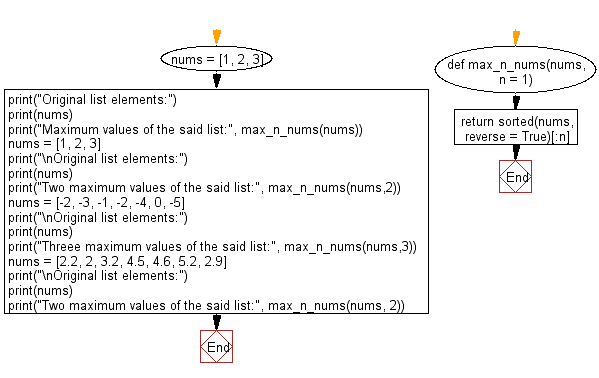﻿ Python: Find the n maximum elements from the provided list - w3resource# Python: Find the n maximum elements from the provided list

## Python List: Exercise - 252 with Solution

Write a Python program to get the n maximum elements from a given list of numbers.

• Use sorted() to sort the list.
• Use slice notation to get the specified number of elements.
• Omit the second argument, n, to get a one-element list.
• If n is greater than or equal to the provided list's length, then return the original list (sorted in descending order).

Sample Solution:

Python Code:

``````def max_n_nums(nums, n = 1):
return sorted(nums, reverse = True)[:n]
nums = [1, 2, 3]
print("Original list elements:")
print(nums)
print("Maximum values of the said list:", max_n_nums(nums))
nums = [1, 2, 3]
print("\nOriginal list elements:")
print(nums)
print("Two maximum values of the said list:", max_n_nums(nums,2))
nums = [-2, -3, -1, -2, -4, 0, -5]
print("\nOriginal list elements:")
print(nums)
print("Threee maximum values of the said list:", max_n_nums(nums,3))
nums = [2.2, 2, 3.2, 4.5, 4.6, 5.2, 2.9]
print("\nOriginal list elements:")
print(nums)
print("Two maximum values of the said list:", max_n_nums(nums, 2))
```
```

Sample Output:

```Original list elements:
[1, 2, 3]
Maximum values of the said list: 

Original list elements:
[1, 2, 3]
Two maximum values of the said list: [3, 2]

Original list elements:
[-2, -3, -1, -2, -4, 0, -5]
Threee maximum values of the said list: [0, -1, -2]

Original list elements:
[2.2, 2, 3.2, 4.5, 4.6, 5.2, 2.9]
Two maximum values of the said list: [5.2, 4.6]
```

Flowchart:## Visualize Python code execution:

The following tool visualize what the computer is doing step-by-step as it executes the said program:

Python Code Editor:

Have another way to solve this solution? Contribute your code (and comments) through Disqus.

What is the difficulty level of this exercise?

Test your Python skills with w3resource's quiz

﻿

## Python: Tips of the Day

Floor Division:

When we speak of division we normally mean (/) float division operator, this will give a precise result in float format with decimals.

For a rounded integer result there is (//) floor division operator in Python. Floor division will only give integer results that are round numbers.

```print(1000 // 300)
print(1000 / 300)```

Output:

```3
3.3333333333333335```